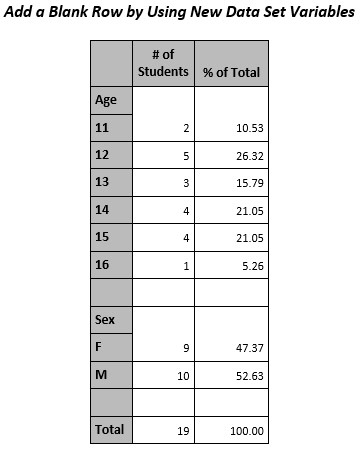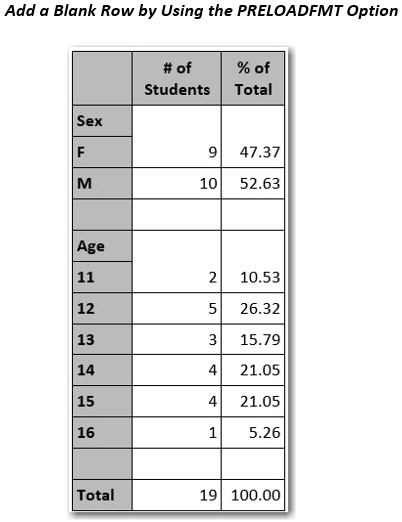SAS Technical Support occasionally receives requests from users who want to insert blank rows into their TABULATE procedure tables. PROC TABULATE syntax does not have any specific options that insert blank rows into the table results.

One way to accomplish this task is explained in SAS Sample 45972, "Add a blank row in PROC TABULATE output in ODS destinations." This sample shows you how to add a blank row between class variables in a PROC TABULATE table.  The sample code creates a new data set variable that you can use in a CLASS statement in the PROC TABULATE step.

In addition to the method that is shown this sample, there are two other methods that you can use to insert a blank row in PROC TABULATE table results. The following sections explain those methods:

### Method 1: Adding a blank row between row dimension variables

This section demonstrates how to add a blank row between row dimension variables.  This method expands on the approach that is used in Sample 45972.

In the following example, additional data-set variables are added to the data set in a DATA step. The BLANKROW variable is used as a class variable, and the variables DUMMY1 and DUMMY2 are used as analysis variables in the PROC TABULATE step. This sample code also uses the AGE and SEX variables from the SASHELP.CLASS data set as class variables in PROC TABULATE.

```/*** Method 1 ***/ data class; set sashelp.class; blankrow=' ';   dummy1=1; dummy2=.; run;   proc tabulate data=class missing; class age sex blankrow; var dummy1 dummy2; table age*dummy1=' ' blankrow=' '*dummy2=' '   sex*dummy1=' ' blankrow=' '*dummy2=' '   all*dummy1=' ',   sum*F=8. pctsum / misstext=' ' row=float;   keylabel sum='# of Students' pctsum='% of Total' all='Total';   title 'Add a Blank Row by Using New Data Set Variables'; run;```

This method produces the result that is shown below.  You can use ODS HTML, ODS PDF, ODS RTF, or ODS EXCEL statements to display the table.### Method 2: Adding blank rows with user-defined formats and the PRELOADFMT option in the CLASS statement

The second method creates user-defined formats and uses the PRELOADFMT option in a CLASS statement in PROC TABULATE.  The VALUE statements that use the NOTSORTED option in the PROC FORMAT step establish the desired order of the results in the TABULATE results. Using the formats and specifying the ORDER=DATA option in the CLASS statement and the PRINTMISS option in the TABLE statement keeps the order requested in the PROC FORMAT VALUE statements and display the blank rows.

```/*** Method 2 ***/ proc format; value \$sexf (notsorted) 'F'='F' 'M'='M' ' '=' ';   value agef (notsorted) 11='11' 12='12' 13='13' 14='14' 15='15' 16='16' .=' ';   value \$sex2f (notsorted default=8) 'F'='F' 'M'='M' ' '='Missing' '_'=' ';   value age2f (notsorted) 11='11' 12='12' 13='13' 14='14' 15='15' 16='16' .=' .' 99=' ';   value mymiss 0=' ' other=[8.2];   run;   proc tabulate data=sashelp.class missing; class sex age / preloadfmt order=data;   table sex age all, N pctn*F=mymiss. / printmiss misstext=' ' style=[cellwidth=2in];   format sex \$sexf. age agef.; /* If there are no missing values for the class */ /* variables, use the formats \$SEXF and AGEF.*/ /* With missing values for the class variables, */ /* use the formats \$SEX2F and AGE2F. */   keylabel N='# of Students' PctN='% of Total' all='Total';   title 'Add a Blank Row by Using the PRELOADFMT Option'; run;```

This method produces the result that is shown below. You can use ODS HTML, ODS PDF, ODS RTF, or ODS EXCEL statements to display the table.If you have any questions about these methods, contact SAS Technical Support. From this link, you can search the Technical Support Knowledge Base, visit SAS Communities to ask for assistance, and contact SAS Technical Support directly.

Share